Courses
Courses for Kids
Free study material
Free LIVE classes
MoreLIVE
Join Vedantu’s FREE Mastercalss

## Introduction to Perimeter of Quadrilateral

Do you see some four-sided objects around you like books, papers, walls, floor, kite, doors, etc.? Do you know the common factor in all of these things? In Geometry, a plane shape with four sides or edges and four corners or vertices is called a quadrilateral. The sum of internal angles of a quadrilateral is 360 degrees. The length of a quadrilateral's boundary as a whole is its perimeter. An irregular or regular four-sided polygon is also referred to as a quadrilateral.## What is the Perimeter of a Quadrilateral?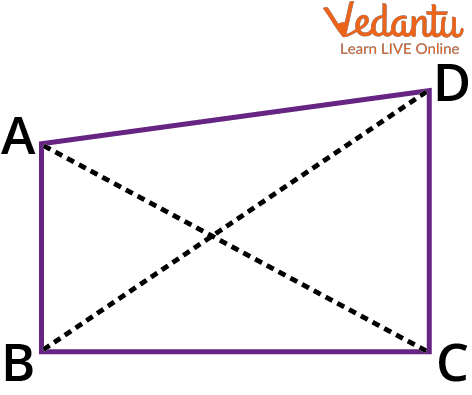What is the Perimeter of a Quadrilateral?

The total length around any two-dimensional shape is called its perimeter.

For example: In the above quadrilateral, the perimeter of the quadrilateral is

ABCD = AB + BC + CD + AD

To find the perimeter of any plane shape, add the length of all the sides. The quadrilateral has four sides. So, add the lengths of four sides to get the perimeter.

## How to Find the Perimeter of a Quadrilateral?

To find the perimeter of a quadrilateral, we need to add all the lengths of four sides, i.e., the perimeter of ABCD Quadrilateral = sum of lengths of all sides = AB +BC + CD + DA.

In other words, if we join all four sides of a quadrilateral such that it forms a line segment, the length of the resultant line segment is called a perimeter. The unit of the perimeter in the S.I system is ‘metre (m)’, and in the CGS system is ‘cm’.

## The Perimeter of Quadrilateral Formula

The following are the perimeter of the quadrilateral formulas or the perimeter equation of quadrilaterals.

### Square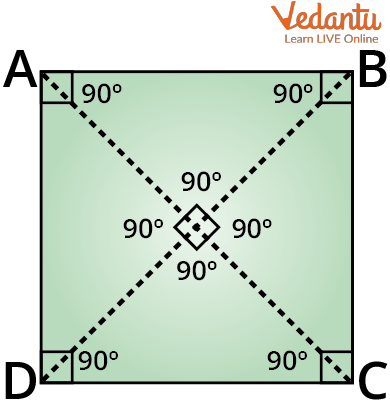A Square

To find the perimeter of the square,

Perimeter of square $A B C D=A B+B C+C D+A D$

As lengths of all sides are equal, i.e. $A B=B C=C D=A D$

Perimeter $=4 \times A B=4 \times$ Length of any side.

### Rectangle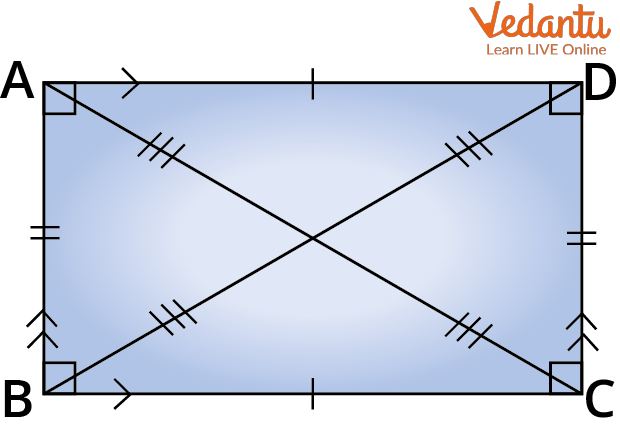A Rectangle

Perimeter of rectangle = AB + BC + CD + AD

As lengths of opposite sides are equal i.e AB = CD = length and BC = AD = breadth

We get, Perimeter of rectangle $=2 \times(A B+B C)$

$=2 \times(C D+A D)$

$=2 \times$ (length + breadth)

### Parallelogram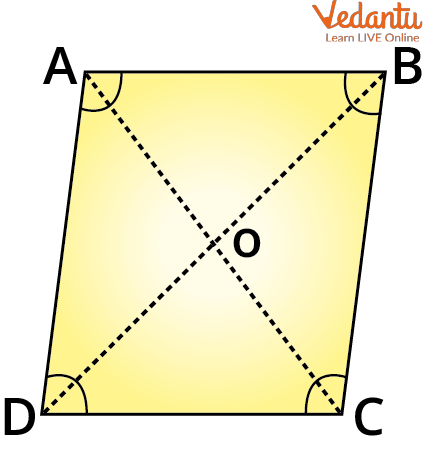A Parallelogram

Perimeter of parallelogram = AB +BC +CD +AD

As lengths of opposite sides are equal i.e., AB =CD= a and BC=AD= b

We get, parallelogram $=2 \times(\mathrm{AB}+\mathrm{BC})$

$=2 \times(C D+A D)=2 \times(a+b)$

## Rhombus

Perimeter of rhombus ABCD = AB +BC +CD +AD

As lengths of all sides are equal i.e. AB = BC = CD = AD = a

Perimeter $=4 \times A B=4 \times \mathrm { Length~of~any~side. } = 4 \times a$

### Kite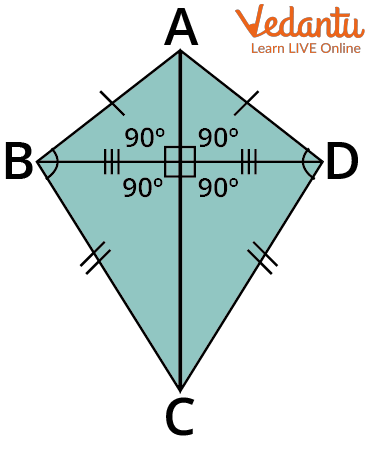A Kite

Here AB = AD=a, BC =CD=b

Perimeter of kite ABCD = AB +BC +CD +AD

$=2 \times(A B+B C)=2 \times(a+b)$

### Trapezium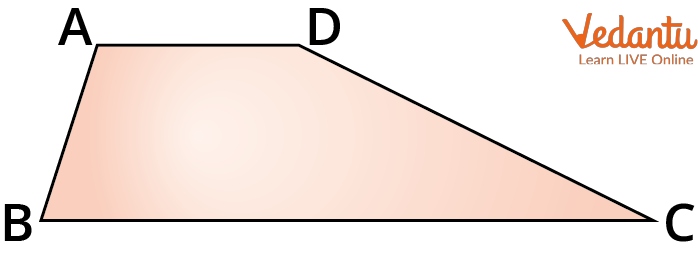A Trapezium

• All sides are unequal

Perimeter of Trapezium ABCD = AB + BC + CD + AD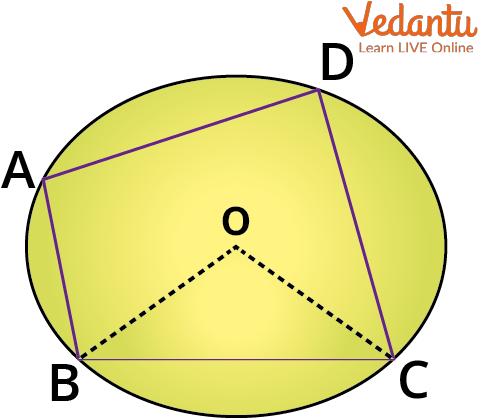A quadrilateral circumscribed in a circle is called a cyclic quadrilateral. It means that all four vertices of a quadrilateral lie in the circumference of the circle.

The perimeter of a cyclic quadrilateral is = AB +BC+CD+DA

Semi perimeter $=\mathrm{s}=\dfrac{AB+BC+CD+AD}{2}$

## Solved Example

Q1. What will be the perimeter of a trapezoid with sides of 2 cm, 5 cm, 8 cm, and 12 cm?

Ans: Given the sides of a are Trapezoid 2 cm, 5 cm, 8 cm, and 12 cm.

Therefore, perimeter of Trapezoid is:

P = (2 cm + 5 cm +8 cm + 12 cm) = 27 cm

## Practice Questions

Q1. Find the perimeter of the ceiling shaped like a rectangle of width 80m and length 30m.

Ans: 220m

Q2. Find the perimeter of the kite shaped like a rhombus of side length 8 m.

Ans: 32m

## Summary

Perimeter of a quadrilateral is given by the sum of lengths of all sides. The perimeter of quadrilateral formula are-

 Quadrilateral Perimeter Formula Square $4 \times$ side Rectangle $2 \times(l+b)$ Parallelogram $2 \times($ sum of adjacent sides $)$ Rhombus $4 \times$ side Kite $2 \times$ sum of lengths of unequal sides) Trapezoid Sum of all sides i.e; $(A B+B C+C D+D A)$
Last updated date: 21st Sep 2023
Total views: 109.2k
Views today: 2.09k

## FAQs on Perimeter of a Quadrilateral

1. What is the formula for the perimeter of an irregular shape?

An irregular figure can be divided into two or more regular shapes like triangles, circles, trapezoids, or rectangles. We can then add the lengths of the sides to obtain the perimeter of an irregular figure.

2. What to do when lengths of all sides are not given to calculate the perimeter?

In such cases, we need to recollect the properties of quadrilaterals with respect to sides to obtain the side lengths that are not given.

For example, if we need to find the perimeter of a rhombus with only one side length given, we need to recollect one of the properties of the rhombus that all its side lengths are equal. So, if we assume one side of the rhombus to be $x$, its perimeter will be $(x+x+x+x) = 4x$

3. How do we find the perimeter of any quadrilateral?

We must sum up the lengths of the rectangle's four sides to get its perimeter. Since there are two of each side, it is easy to accomplish this by simply adding the length and width and multiplying the result by two.